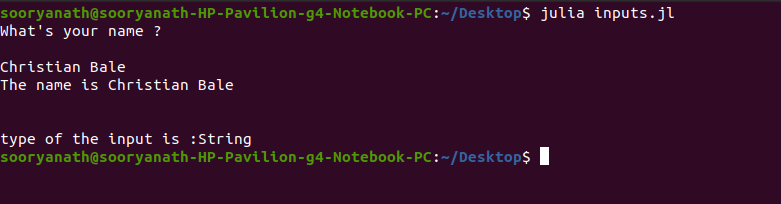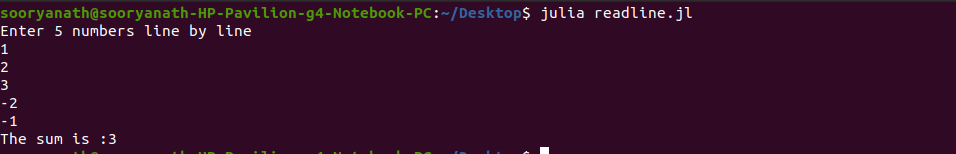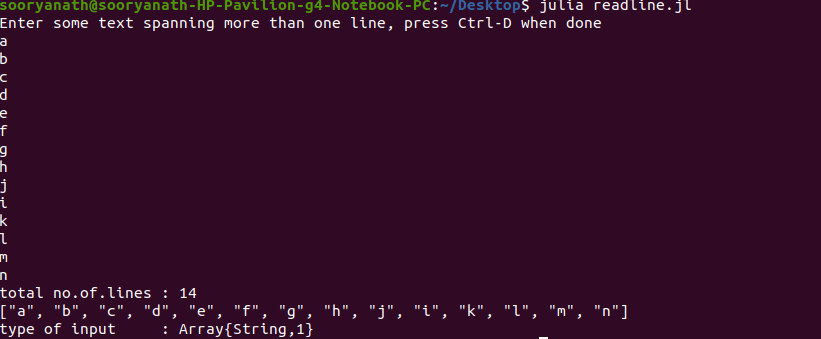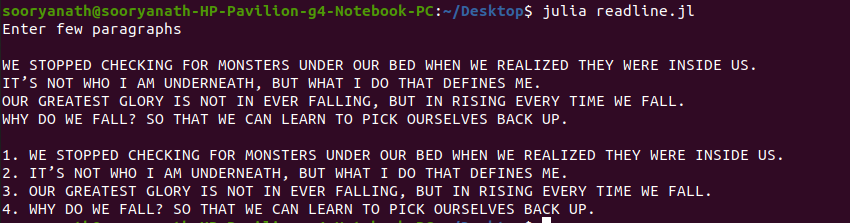# Taking Input from Users in Julia

• Last Updated : 28 Jul, 2020

Reading user input is a way of interaction between the program and the user. User inputs are necessary in case of testing a piece of code with varying and legitimate inputs.

Reading user inputs from console in Julia can be done through inbuilt I/O methods like :

#### Using readline() Function

`readline()` method reads a line of text from the input stream (STDIN) until a ‘\n’ (newline) character is encountered. The input is read as String data.

Syntax:

```s = readline()
```

Example 1:
In the below example, user input is obtained using the `readline()` method. We can prompt the user with the help of a print statement prior. It is to be noted that readline method reads the input into a string data type.

 `# Julia program to take input from user`` ` `# prompt to input``print``(``"What's your name ? \n\n"``) `` ` `# Calling rdeadline() function``name ``=` `readline()`` ` `println(``"The name is "``, name)``print``(``"\n\n"``)`` ` `# typeof() determines the datatype.``println(``"Type of the input is: "``, typeof(name)) `

Output :Example 2: Reading numerical data types from console

The below example demonstrates how to read inputs as numbers and make use of them in further computations. This is done using the `parse()` method, using which we can convert a numeric string(Float or Int) into a numerical value.

 `# Julia program to calculate sum of ``# 5 integers obtained from console/user input`` ` `result ``=` `0`` ` `# Prompt to enter``println(``"Enter 5 numbers line by line"``)`` ` `# Taking Input from user``for` `number ``in` `1``:``5` ` ` `   ``num ``=` `readline()``   ``num ``=` `parse(Int64, num) ``   ``global` `result``+``=` `num  `` ` `end`` ` `println(``"The sum is :"``, result)`

Output:#### Using readlines() Function

`readlines()` method is used to read N lines of text input from the console. N lines are stored as the entries of a one-dimensional String array. Here lines must be delimited with newline character or by pressing the “ENTER” key. To stop taking Input press Ctrl-D.

Syntax:

```lines = readlines()
```

Example 1: Reading N lines of input from STDIN
In the below example N-lines of input are obtained and stored within a 1-D String Array. We can access the desired line using it’s index.

 `# Julia program to take ``# multi-lined input from user`` ` `line_count ``=` `0`` ` `println(``"Enter multi-lined text, press Ctrl-D when done"``)`` ` `# Calling readlines() function``lines ``=` `readlines()`` ` `# Loop to count lines``for` `line ``in` `lines``   ` `   ``global` `line_count ``+``=` `1``  ` `end`` ` `println(``"total no.of.lines : "``, line_count)`` ` `println(lines)`` ` `# Getting type of Input values``println(``"type of input: "``, typeof(lines))`

Output:### Example 2

In the below example we input N lines and display the entries by prefixing with their entry numbers.

 `line_number ``=` `1`` ` `println(``"Enter few paragraphs"``)`` ` `print``(``"\n"``)`` ` `lines ``=` `readlines()`` ` `println()`` ` `for` `line ``in` `lines``   ` `   ``println(line_number, ``". "``, line)``   ``global` `line_number ``+``=` `1``  ` `end`

Output:My Personal Notes arrow_drop_up